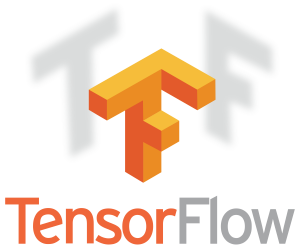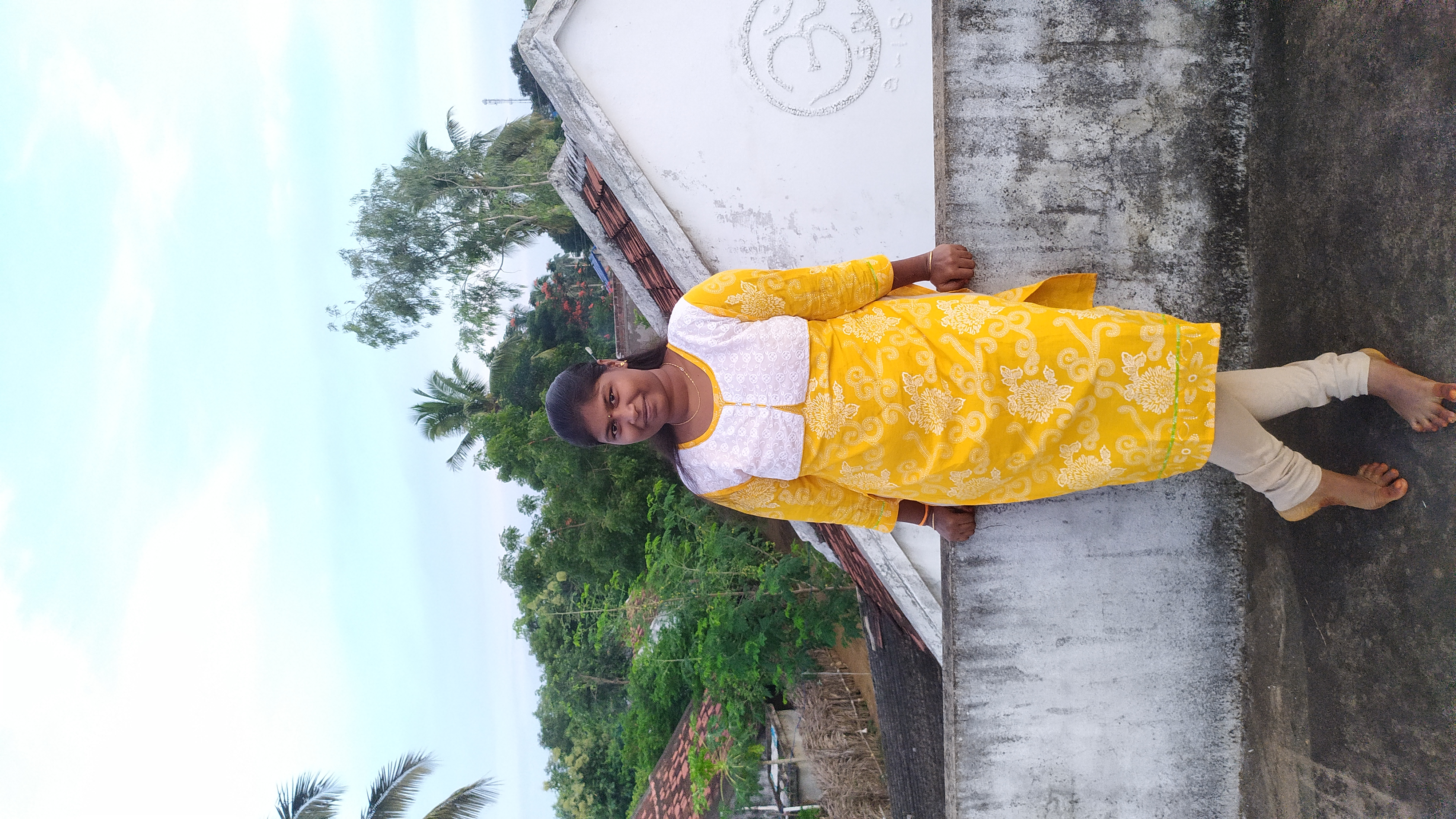Amrutha K — Published On July 13, 2022 and Last Modified On July 25th, 2022

This article was published as a part of the Data Science Blogathon.

## Introduction

In this article, we will see how to build an application that can recognize digits that are written by hand. The application will recognize our handwriting. Nowadays, technology has increased tremendously, and the computer can recognize anything. Here there are two categories for recognizing our writing. One recognizes alphabets, and the other is digits. This article will show how to build an application to recognize hanSource: Dreamstime.com

#### Requirements

Now let’s see the required libraries to build our handwritten digit recognition application. Import the following libraries. Before importing, these libraries should be reinstalled on your desktop. If any of them are not installed, install them using pip install.

Like,

`pip install tensorflow`

Python: We use python as the programming language to build the application

Tensorflow: Tensorflow is an open-source library, and we use TensorFlow to train and develop machine learning models.

Keras: It is also an open-source software library and a high-level TensorFlow API. It also provides a python interface for Artificial Neural Networks.

Tkinter: Tkinter is a fantastic package that provides a way to create Graphical User Interfaces (GUIs). We use Tkinter to provide a Graphical User Interface for our application.

PIL: PIL stands for Python Imaging Library, which allows the python interpreter to edit the images.

NumPy: We all know about the NumPy library, a basic standard library used to work with arrays.

win32gui: we also need to install win32gui to work with graphical user interfaces.#### Dataset

To build this application, we use the MNIST dataset. This dataset contains images of digits from 0 to 9. All these images are in greyscale. There are both training images and testing images. This dataset contains about 60000 training images which are very large and about 10000 testing images. All these images are like small squares of 28 x 28 pixels in size. These are handwritten images of individual digits. These images look like the following.

Source: Machine Learning Mastery

Let us see the implementation of the application.

#### Importing Libraries

Import all the required libraries before writing any code. In the beginning, I had already mentioned all the requirements for building the application. So import those libraries. From PIL library import ImageGrab and Image.

```import numpy as np
from tkinter import *
import tkinter as tk
import win32gui
from PIL import ImageGrab, Image```

## Building Model using Tensorflow

To build the model first we need to import some libraries from TensorFlow Keras. We have to import keras from TensorFlow and then import the dataset that we are going to use to build our application now. That is the MNIST dataset. Then import the sequential model, and some layers like Dense, Dropout, Flatten Conv2D, MaxPooling2D, and finally import the backend.

After importing all the required libraries, split the dataset into train and test datasets. Reshape training set of x and testing set of x. The next step is to convert class vectors to binary class matrices.

```from tensorflow import keras
from tensorflow.keras.datasets import mnist
from tensorflow.keras.models import Sequential
from tensorflow.keras.layers import Dense, Dropout, Flatten
from tensorflow.keras.layers import Conv2D, MaxPooling2D
from tensorflow.keras import backend as K

(x_train, y_train), (x_test, y_test) = mnist.load_data()
print(x_train.shape, y_train.shape)
x_train = x_train.reshape(x_train.shape, 28, 28, 1)
x_test = x_test.reshape(x_test.shape, 28, 28, 1)
input_shape = (28, 28, 1)
y_train = keras.utils.to_categorical(y_train, 10)
y_test = keras.utils.to_categorical(y_test, 10)
x_train = x_train.astype('float32')
x_test = x_test.astype('float32')
x_train /= 255
x_test /= 255
print('x_train shape:', x_train.shape)
print(x_train.shape, 'train samples')
print(x_test.shape, 'test samples')```

## Training the Model on Tensorflow

Our next step is to train the model. For that define batch size, the number of classes, and the number of epochs that you want to train your model. next add some layers to the sequential model which we imported before. Then compile the model using categorical cross-entropy loss function, Adadelta optimizer, and accuracy metrics. Finally using x_train,y_train, batch size, epochs, and all train the model. Then save it for later.

```batch_size = 128
num_classes = 10
epochs = 30
model = Sequential()
hist = model.fit(x_train, y_train,batch_size=batch_size,epochs=epochs,verbose=1,validation_data=(x_test, y_test))
print("The model has successfully trained")
score = model.evaluate(x_test, y_test, verbose=0)
print('Test loss:', score)
print('Test accuracy:', score)
model.save('mnist.h5')
print("Saving the model as mnist.h5")```

## Predicting Digit

Now we have to write some code for predicting the digit that we have written. For that define a function predict_class where you provide an image as an argument. First, resize it into the required pixels. Convert the image into grayscale which was previously in RGB. Then reshape and normalize it. Finally, predict the image using predict method.

```model = load_model('mnist.h5')
def predict_digit(img):
#resize image to 28x28 pixels
img = img.resize((28,28))
#convert rgb to grayscale
img = img.convert('L')
img = np.array(img)
img = img.reshape(1,28,28,1)
img = img/255.0
img = 1 - img
#predicting
res = model.predict([img])
return np.argmax(res), max(res)```

## Building Application

Let us see how to build a user-friendly GUI application for it. We use Tkinter for it. Here we create some space for the user to actually draw the digit and then provide two buttons Recognize and clear. Recognize button is to recognize the digit that is written on the given space and the clear button is to clear the writings on it. Finally, run the main loop to run the application.

```class App(tk.Tk):
def __init__(self):
tk.Tk.__init__(self)
self.x = self.y = 0
# Creating elements
self.canvas = tk.Canvas(self, width=400, height=400, bg = "white", cursor="cross")
self.label = tk.Label(self, text="Thinking..", font=("Helvetica", 48))
self.btn_classify = tk.Button(self, text = "Recognise", command =self.classify_handwriting)
self.clear_button= tk.Button(self, text = "Clear",command = self.clear_all)
self.canvas.bind("", self.draw_lines)
def clear_all(self):
self.canvas.delete("all")
def classify_handwriting(self):
HWND = self.canvas.winfo_id()
rect = win32gui.GetWindowRect(HWND)
im = ImageGrab.grab(rect)
digit, acc = predict_digit(im)
self.label.configure(text= str(digit)+', '+ str(int(acc*100))+'%')
def draw_lines(self, event):
self.x = event.x
self.y = event.y
r=8
self.canvas.create_oval(self.x-r, self.y-r, self.x + r, self.y + r, fill='black')
app = App()
mainloop()```

## Results

When you run the application a window will pop up where you can write the digit. And next, when you click on recognize button, it will recognize the digit you have written with the probability percentage showing how exactly the digit matches with the original one. Here I have written digit 1 and it recognized it as 1 with 17% accuracy.

## Conclusion to Tensorflow

Computers are smarter and fast nowadays. These are well developed in such a way that they are able to do most of the intelligence tasks done by human beings. Handwriting recognization is one among them. In that, we have learned how to build an application to recognize the digits. We have also used Tkinter to provide the Graphical User Interface and built the application. You can also change some values, fonts, and colors and can create a better one than this. You can play around with it to get some more knowledge.

• Thus we have seen how to build an application to recognize handwritten digits.
• How to use the MNIST dataset
• Building, training, and testing of the model
• Built GUI using Tkinter

Hope you guys found it useful.